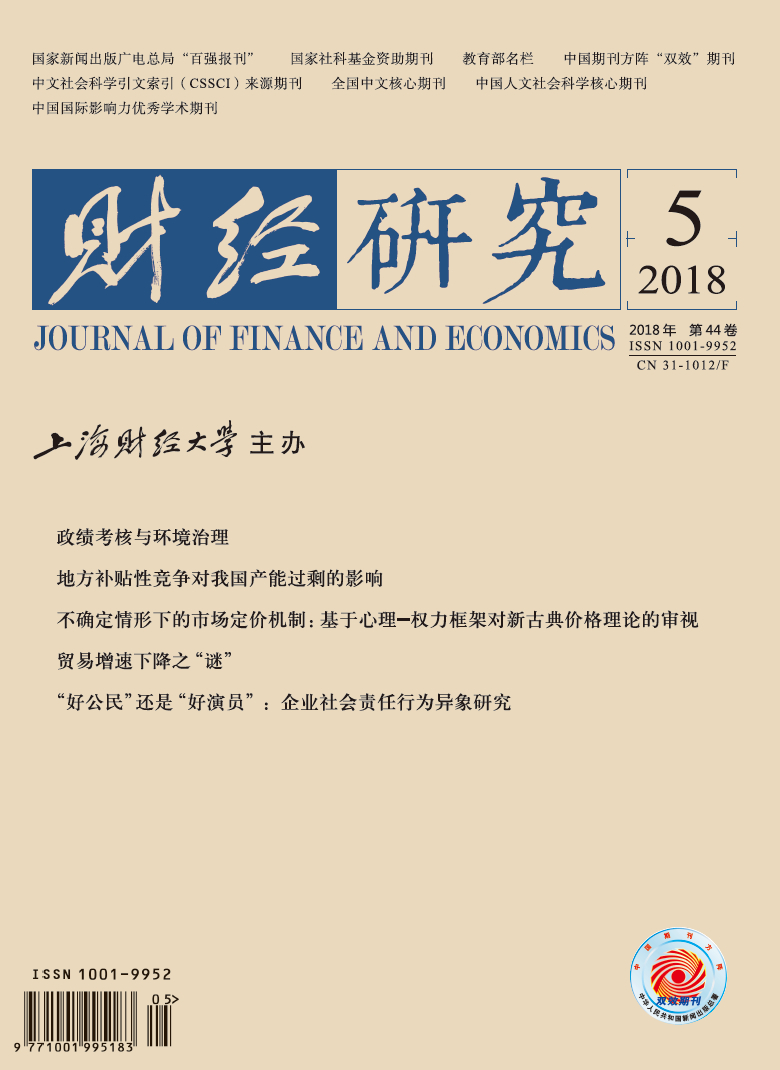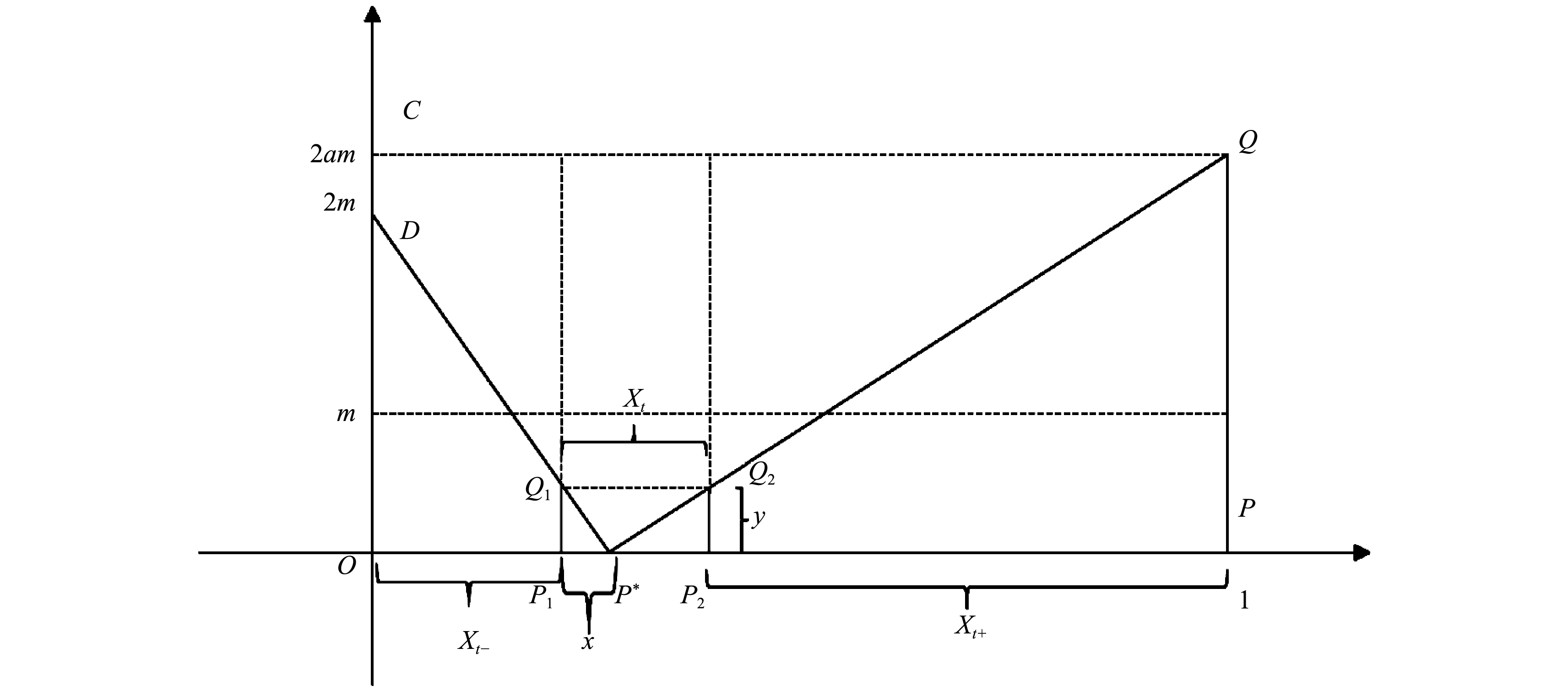﻿ 贸易增速下降之“谜”——基于全球价值链分工视角的解释
 财经研究2018, Vol. 44Issue (5): 83-960

#### 文章信息财经研究2018年44卷第5期

Zhou Jing, Dai Xiang, Liu Meng.

The “mystery” of declining trade growth: interpretation from the perspective of global value chain division of labor

Journal of Finance and Economics, 2018, 44(5): 83-96.

### 文章历史《财经研究》
2018第44卷第5期

1. 安徽财经大学 国际经济贸易学院，安徽 蚌埠 233030;
2. 南京审计大学 经济学院，江苏 南京 211815;
3. 东南大学 经济管理学院，江苏 南京 211189

The “Mystery” of Declining Trade Growth: Interpretation from the Perspective of Global Value Chain Division of Labor
Zhou Jing1, Dai Xiang2, Liu Meng3
1. School of International Economics and Trade，Anhui University of Finance and Economics，Bengbu 233030，China;
2. School of Economics，Nanjing Audit University，Nanjing 211815，China;
3. School of Economics and Management，Southeast University，Nanjing 211189，China
Key words: global value chain    declining trade growth    dynamic evolution

WTO的统计数据显示，近年来的全球贸易增速已经远低于1983—2008年的年均增幅（年均6%的增长率），并且与以往远高于经济增速的情形相比，近年来的全球贸易增速已与全球经济增速持平，甚至落后于全球GDP增速。有学者将这种现象称为全球贸易发展的“新常态”。这种变化引起了理论研究者和实际工作者的深刻担忧，因为传统的经典贸易理论表明，外贸是驱动经济增长的“发动机”，所以外贸增速下降甚至出现负增长，将意味着外贸无法继续发挥其带动经济发展的作用，甚至有可能会出现所谓的“负”贡献。值得注意的是，一方面，全球贸易增速变化与全球经济增速变化打破了过去几十年来的惯常关系，即全球贸易增速从远远高于全球GDP增速下降到与后者基本持平，甚至落在后者之下；另一方面，也是更为重要的，2008年金融危机以来的全球贸易增速逐年显著下滑的经济现实，是需求减弱效应、信贷紧缩效应和贸易保护主义抬头效应等不同理论视角所不能完全解释的，因为按照上述理论预期，全球主要国家联手采取凯恩斯式宏观刺激政策，将在很大程度上遏制上述三大负面效应，但现实是全球贸易并未带来恢复性增长。可见，全球贸易增速表现出的如此变化逻辑不仅超出了正常预期，而且在现有理论研究中也未能找到充分的解释。为此，本文不妨将这一现象称为全球贸易增速变化之“谜”。那么，究竟是什么因素导致了包括中国在内的全球贸易“失速”呢？对此，学术界针对2008年危机后的全球贸易崩溃进行了广泛探讨，在此基础上所形成的一系列代表性观点具有重要启发意义，如全球经济疲软造成的需求不足、危机后全球贸易保护主义的兴起（Anderson等，2015）、进出口企业融资受阻（佟家栋和刘竹青，2014）等。客观而论，目前全球贸易下滑的确是由多种因素造成的。由于关注的侧重点不同，不同学者基于不同视角观察所得的结论无疑均具有重要启示。但是，2008年危机冲击期间全球贸易“大崩溃”与当前全球贸易失速之“谜”并非完全等同，因此，针对前者研究所取得的成果还不能很好地解释当前的现象。综合来看，已有研究文献所形成的一个基本共识是，前一轮包括中国在内的全球贸易高速增长，实质上是得益于全球价值链分工的深入演进。虽然当前国际分工的主导形态仍然是全球价值链，但是，在同样的分工形态下为何会出现“高速”和“失速”的前后巨大反差？这可能与价值链本身动态演进所呈现的阶段性特征有关。

20世纪70年代中后期以来全球贸易的迅猛发展，引起了学者们对贸易增长影响因素的研究兴趣。综合来看，较为一致的观点是，这主要得益于全球经济的繁荣稳定、贸易和投资自由化的深入推进以及在此背景下全球价值链分工的深入发展。一方面，世界经济繁荣发展为全球贸易高速增长奠定了基础并提供了广阔的国际市场需求空间（Berman等，1994）；另一方面，贸易与投资自由化则为贸易高速增长提供了便利和制度保障（Krugman等，1995），突出表现为WTO主导下全球贸易规则的顺利推行，以及各种区域经济一体化组织的快速发展。而尤为重要的是，正如众多学者所指出的，经济增长、以关税降低为主要表现的贸易便利性水平提高、运输成本的下降等因素，只能解释全球贸易高速增长中的2/5，而其余部分则与全球价值链为主导的国际分工形态有关（Hummels等，2001；刘志彪等，2006），即全球价值链分工背景下中间产品的多次跨境流动“放大”了贸易增速。

${U_A} = \max {\left[ {\left( {X_A^{self} + X_A^{buy}} \right)Y_A^{self}} \right]^{\displaystyle{\textstyle{\frac{1}{2}}}}}$ ${U_B} = \max {\left( {X_B^{self}Y_B^{buy}} \right)^{\displaystyle{\textstyle{\frac{1}{2}}}}}$

$s.t.X_A^{self} = \displaystyle\frac{{{l_{AX}}}}{m}$ $X_1^{Bself} = \displaystyle\frac{{{l_B}}}{{am/{k^2}}}$ $X_{1 + }^{Asell} = \displaystyle\frac{{{l_{A{X_{1 + }}}}}}{{m/k}} = X_{1 + }^{Bbuy} = X_1^{Bself}$ $Y_A^{self} + Y_A^{sell} = \displaystyle\frac{{{l_{AY}}}}{n}$ $X_B^{self} + X_B^{sell} = \displaystyle\frac{{{l_B}}}{{am/{k^2}}}$ ${l_{AX}} + {l_{A{X_{1 + }}}} + {l_{AY}} = {l_A}\text{，}$ $X_B^{sell} m = X_{1 + }^{Bbuy} \displaystyle\frac{{k - 1}}{k}m + Y_B^{buy} n$

 ${v_1} = \frac{{\left( {k + \Delta k} \right)\left( {2k + 2\Delta k - 1} \right) - k\left( {2k - 1} \right)}}{{k\left( {2k - 1} \right)}} = \frac{{\Delta k\left( {4k + 2\Delta k - 1} \right)}}{{k\left( {2k - 1} \right)}}$ (1)图 1 B国生产产品X各阶段所需的有效劳动投入

${U_A} = \max {\left( {X_A^{self}Y_A^{self}} \right)^{\displaystyle{\textstyle{\frac{1}{2}}}}}$ ${U_B} = \max {\left( {X_B^{buy}Y_B^{buy}} \right)^{\displaystyle{\textstyle{\frac{1}{2}}}}}$

$s.t.X_A^{self} + X_A^{sell} = \displaystyle\frac{{{l_{AX}}}}{m} + \displaystyle\frac{{{l_{A{X_{\bar t}}}}}}{{\left( {k - 1} \right)m/k}}$ $X_t^{Bsell} = \displaystyle\frac{{{l_B}}}{{{B_t}}}$ $Y_A^{self} + Y_A^{sell} = \displaystyle\frac{{{l_{AY}}}}{n}$

$X_B^{buy} m + Y_B^{buy} n = X_t^{Bsell} \displaystyle\frac{m}{k}$ $X_t^{Bsell} = \displaystyle\frac{{{l_{A{X_{\bar t}}}}}}{{\left( {k - 1} \right)m/k}}$ ${l_{AX}} + {l_{A{X_{\bar t}}}} + {l_{AY}} = {l_A}$

（一）变量选取与模型设定

 $GV{L_j} = \sum\limits_i^n {{w_{ij}}GV{L_{ij}}}$ (2)

 $GVL = \sum\limits_j^m {{w_j}GV{L_j}}$ (3)

 $E{X_{i,t}} = {\alpha _0} + {\alpha _1}GV{L_t} + {\alpha _2}GVL_t^2 + {\alpha _{}}GV{P_{i,t}} + \beta {C_{i,t}} + {\mu _t} + {\nu _i} + {\varepsilon _{i{\rm{,}}t}}$ (4)

（二）数据来源及说明

（一）基准模型的GLS和工具变量法估计结果

 （1）固定效应 （2）固定效应 （3）固定效应 （4）固定效应 （5）工具变量 （6）工具变量 （7）工具变量 （8）工具变量 GVL1 0.041**（2.37） 0.041**（2.74） 0.043**（2.12） 0.043**（2.65） （GVL1）2 −0.018**（−2.15） −0.017*（−2.03） −0.018*（−1.95） −0.018**（−2.23） GVL2 0.248***（3.48） 0.245**（3.44） 0.255**（2.57） 0.219**（2.54） （GVL2）2 −0.114***（−2.51） −0.112**（−2.76） −0.117**（−2.68） −0.110***（−3.54） GVP 0.013**（2.12） 0.013**（2.05） 0.014**（2.45） 0.018**（2.09） 0.011**（2.47） 0.012**（2.43） 0.013**（2.09） 0.013**（2.09） GDPR 0.025**（2.65） 0.023**（2.32） 0.024**（2.36） 0.023**（2.31） TF 1.328*（1.96） 1.313*（1.94） 1.343*（1.98） 1.274*（1.98） FDI 0.004*（1.96） 0.003*（1.95） 0.005*（1.91） 0.004**（2.70） LnREER −0.049（−1.30） −0.041（−1.07） −.0451（−1.16） −.0432（−1.12） WAGE −0.003*（−1.89） −0.004*（−1.93） −0.003**（−2.34） −0.004**（−2.31） IN 0.086**（2.21） 0.074*（1.95） 0.080**（2.92） 0.082**（2.02） 常数项 −0.152（−0.73） 0.189**（2.52） 0.189**（2.52） 0.619（1.21） −0.063（−0.64） −0.077（−0.80） 0.972**（2.43） 0.923**（2.42） 样本数 680 680 680 680 640 640 640 640 R2 0.313 0.366 0.393 0.402 0.332 0.323 0.385 0.399 hausman 93.671(0.00) 102.362(0.00) 108.152(0.00) 99.372(0.00) 不足识别检验 34.152（0.00） 36.336（0.00） 29.281（0.00） 28.631（0.00） 弱识别检验 22.5316（0.05） 22.213（0.05） 26.519（0.01） 26.283（0.01） 过度识别检验 2.169（0.35） 3.531（0.16） 0.597（0.69） 1.382（0.49） 注：列（5）—列（8）采用的工具变量为GVL和GVP变量的一阶滞后项；弱工具变量检验括号里的值为Cragg-Donald Wald F的10%水平标准值；系数值括号里为t值或z值，***、**、*和#分别表示1%、5%、10%和15%的统计显著水平。下同。

（二）GMM估计结果及进一步稳健性检验

 $E{X_{i,t}} = {\alpha _0} + {\alpha _1}E{X_{i,t - 1}} + {\alpha _2}GV{L_t} + {\alpha _3}GVL_t^2 + {\alpha _3}GV{P_{i,t}} + \beta {C_{i,t}} + {\mu _t} + {\nu _i} + {\varepsilon _{i{\rm{,}}t}}$ (5)

 （1） （2） （3） （4） EX（−1） 0.058**（2.34） 0.067**（2.55） 0.052**（2.20） 0.053**（2.22） GVL1 0.039**（2.18） 0.038**（2.56） （GVL1）2 −0.017**（−2.01） −0.016*（−1.96） GVL2 0.249***（3.15） 0.246**（3.67） （GVL2）2 −0.114***（−3.07） −0.113**（−2.55） GVP 0.014**（2.35） 0.013**（2.16） 0.013**（2.51） 0.018**（2.33） GDPR 0.023**（2.18） 0.024**（2.63） TF 1.331*（1.93） 1.323**（2.61） FDI 0.003*（1.98） 0.004*（1.93） LnREER −0.043（−1.28） −0.042（−1.18） WAGE −0.003*（−1.91） −0.004*（−1.95） IN 0.083**（2.15） 0.081*（2.33） 常数项 −0.052（−0.55） 0.141（1.48） 1.287**（2.38） 1.351（0.641） 样本数 600 600 600 600 时间固定效应 控制 控制 控制 控制 国家固定效应 控制 控制 控制 控制 Wald检验 25.561（0.00） 26.184（0.00） 34.212（0.00） 35.915（0.00） Sargan检验 26.132（0.26） 23.356（0.49） 29.336（0.25） 29.375（0.24） AR（1）检验p值 0.031 0.273 0.067 0.217 AR（2）检验p值 0.853 0.692 0.841 0.793